# We roll a fair 8-sided die five times. (A fair 8-sided die is equally likely to...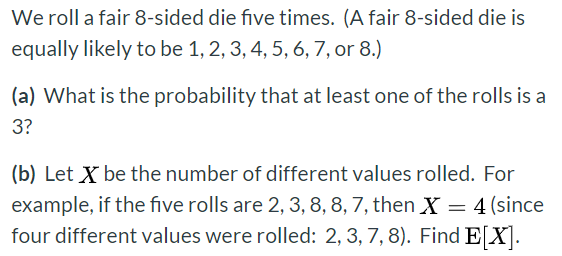We roll a fair 8-sided die five times. (A fair 8-sided die is equally likely to be 1, 2, 3, 4, 5, 6, 7, or 8.) (a) What is the probability that at least one of the rolls is a 3? (b) Let X be the number of different values rolled. For example, if the five rolls are 2, 3, 8, 8, 7, then X = 4 (since four different values were rolled: 2,3,7,8). Find E[X].

a) Probability that at least one of the rolls is a 3= 1 - Probability that none of the rolls is a 3

Probability of getting a 3= 1/8

Probability of not getting a 3= 1-1/8 = 7/8

Since each roll is independent therefore probability of not getting a 3 in any 5 rolls=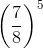Therefore probability of getting at least one of the rolls as 3=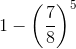b) The values of X can be from the set X = {1,2,3,4,5}

Total sample space size=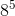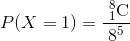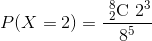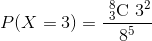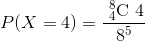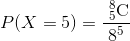Now,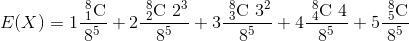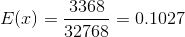##### Add Answer of: We roll a fair 8-sided die five times. (A fair 8-sided die is equally likely to...
Similar Homework Help Questions
• ### we repeatedly roll a fair 8-sided die six times and suppse X is the number of...

we repeatedly roll a fair 8-sided die six times and suppse X is the number of different values rolled. Find E[x] and E[Y]

• ### Question 3 3 pts Matching problem [Choose] You roll a fair six-sided die 500 times and...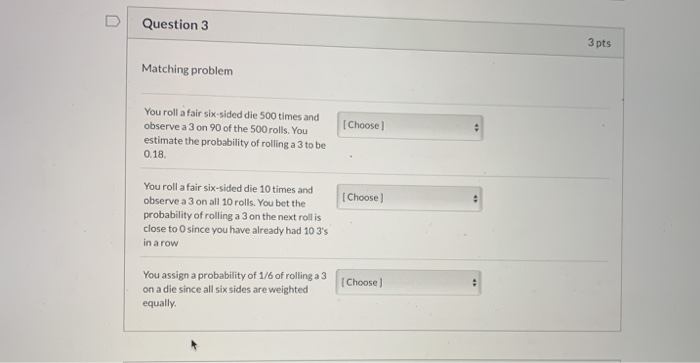Question 3 3 pts Matching problem [Choose] You roll a fair six-sided die 500 times and observe a 3 on 90 of the 500 rolls. You estimate the probability of rolling a 3 to be 0.18 Choose) You roll a fair six-sided die 10 times and observe a 3 on all 10 rolls. You bet the probability of rolling a 3 on the next rollis close to O since you have already had 10 3's in a row You assign...

• ### 6. A fair six sided die is rolled three times. Find the probability that () all...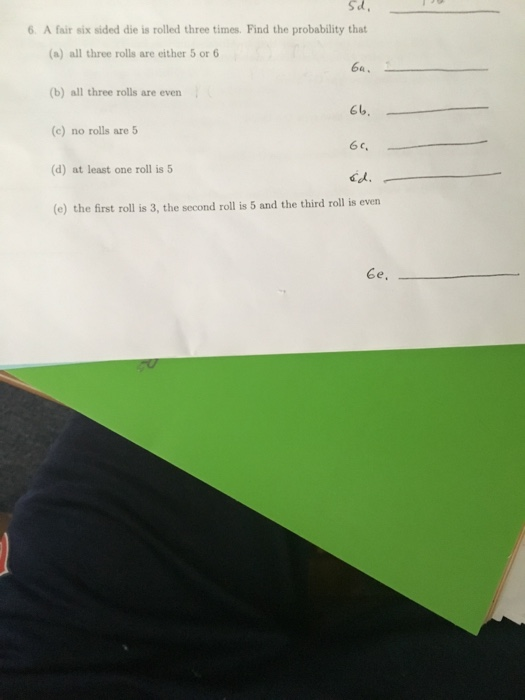6. A fair six sided die is rolled three times. Find the probability that () all three rolls are either 5 or 6 (6) all three rolls are even (c) no rolls are 5 (d) at least one roll is 5 (e) the first roll is 3, the second roll is 5 and the third roll is even

• ### You roll a fair six-sided die 5 times. What is the probability that EXACTLY one of...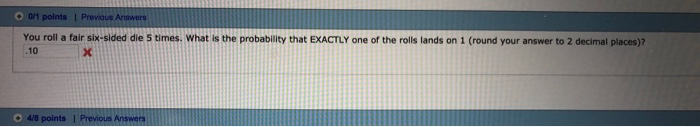You roll a fair six-sided die 5 times. What is the probability that EXACTLY one of the rolls lands on 1 (round your answer to 2 decimal places)? 10 4/8

• ### Suppose I asked you to roll a fair six-sided die 6 times. You have already rolled...

Suppose I asked you to roll a fair six-sided die 6 times. You have already rolled the die for 5 times and six has not appeared ones. Assuming die rolls are independent, what is the probability that you would get a six in the next roll? 1/6 1/2 5/6 0 1

• ### A fair 6-sided die rolled 5 times. what is the probability that at least one of...

A fair 6-sided die rolled 5 times. what is the probability that at least one of the rolls is 2

• ### Sampling Statistics, fair six-sided dieRoll a fair six-sided dine n times, and let 'X bar' denote the average of the n rolls.a) If n=50, give the approximate distribution of 'X bar' and its parameter(s).b) If n=50, find the probability that 'X bar' is between 3 and 4.c) How many times must you roll the die to be at least 90% certain that the average 'X bar' will fall between 3 and 4? That is, find the smallest n suchthat

• ### you repeatedly roll an ordinary six sided die five times. Let X equal the number of...

you repeatedly roll an ordinary six sided die five times. Let X equal the number of times you roll the die. For example (1,1,2,3,4) then x =4 Find E[X]

• ### If a fair six sided die is rolled three times....

1) If a fair six-sided die is rolled three times and the number of spots on the resulting upward face is observed for each roll, what is the probability that the 2ndnumber observed will be greater than the 1st number observed and the 3rd number observed will be equal to the sum of the first two numbers observed? (Make the usualassumptions concerning fair dice.)(Hint: Noting that we can work with a sample space having equally-likely outcomes, the probability can be...

• ### Problem 5. A lopsided six-sided die is rolled repeatedly, with each roll being independent. The probabil-...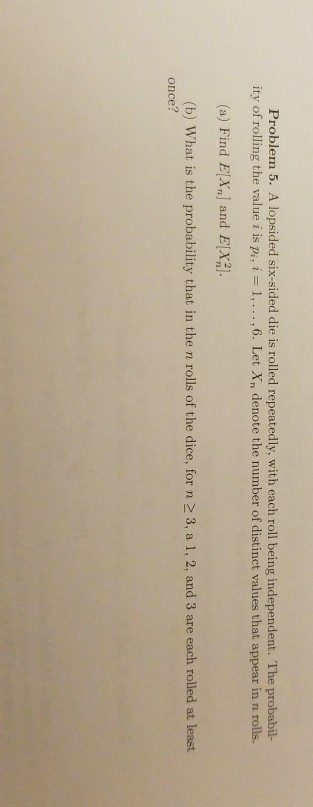Problem 5. A lopsided six-sided die is rolled repeatedly, with each roll being independent. The probabil- ity of rolling the value i is Pi, i = 1, … ,6. Let Xn denote the number of distinct values that appear in n rolls. (a) Find E|X, and E21 (b) What is the probability that in the n rolls of the dice, for n 2 3, a 1, 2, and 3 are each rolled at least once?

Free Homework App